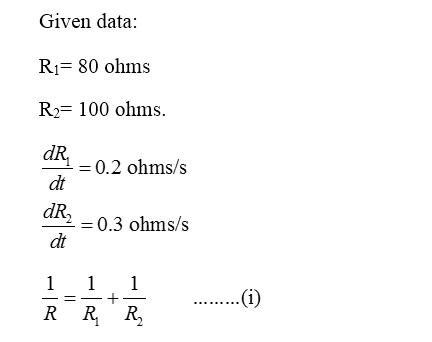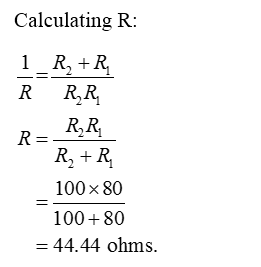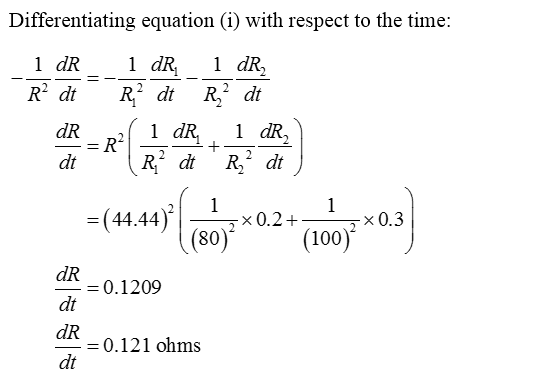# If two resistors with resistances R1 and R2 are connected in parallel, then the total resistance, R, measured in ohms (Omega sign) is given by:(1/R)=(1/R1)+(1/R2). If R1 is increasing at a rate of .2 ohms/s and R2 is increasing at a rate of .3 ohms/s, how fast is R changing when R1= 80 ohms and R2= 100 ohms.

Question
43 views

If two resistors with resistances R1 and R2 are connected in parallel, then the total resistance, R, measured in ohms (Omega sign) is given by:

(1/R)=(1/R1)+(1/R2). If R1 is increasing at a rate of .2 ohms/s and R2 is increasing at a rate of .3 ohms/s, how fast is R changing when R1= 80 ohms and R2= 100 ohms.

check_circle

Step 1Step 2Step 3...

### Want to see the full answer?

See Solution

#### Want to see this answer and more?

Solutions are written by subject experts who are available 24/7. Questions are typically answered within 1 hour.*

See Solution
*Response times may vary by subject and question.
Tagged in